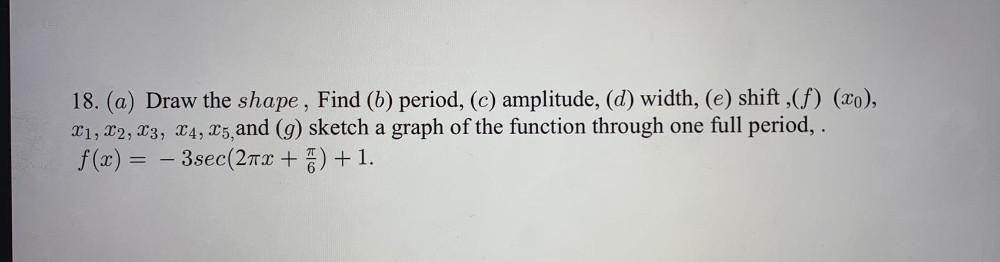Question:

# Draw the shape, Find (b) period, (c) amplitude, (d) width, (e) shift (f) (x₀), x₁, x₂, x₃, x₄, x₅, and (g) sketch a graph of the function through one full period, . f(x) = – 3 sec(2πx + π/6) + 1.Draw the shape, Find (b) period, (c) amplitude, (d) width, (e) shift (f) (x₀), x₁, x₂, x₃, x₄, x₅, and (g) sketch a graph of the function through one full period, . f(x) = – 3 sec(2πx + π/6) + 1.